## How to calculate age (convert birthdate to age) quickly in Excel?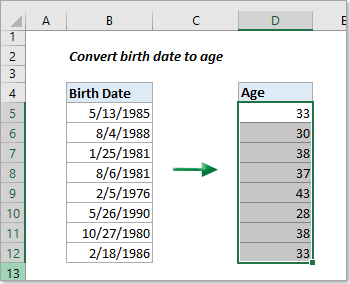Imagine you're working with a list of birth dates in Excel and need to show their precise current ages. How can you efficiently achieve this? This article provides tips for easily calculating age from a date of birth in Excel.

#### Calculate age from date of birth with formulas

In this section, we've outlined various formulas to calculate age from the date of birth under different scenarios:

### (AD) Calculate age in Excel with Kutools in a few clicks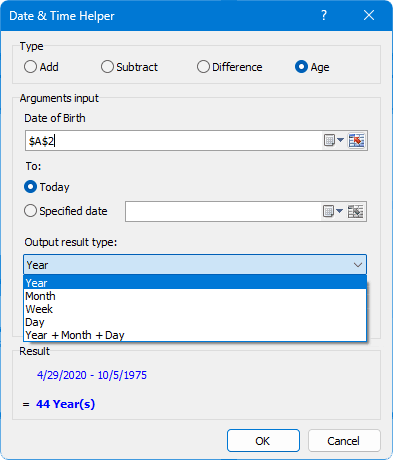Looking to effortlessly calculate ages up to today or any given date and present them in diverse formats such as years, months, weeks, days, or a year + month + day combination? Kutools for Excel simplifies this task! No need for manual formula entries – Kutools streamlines the entire process, offering a user-friendly solution for all Excel users. Discover more here: Easily calculate age from date of birth without remembering formulas. Besides calculating ages, Kutools for Excel offers 300 more features for Excel. Try them all in a 30-day free trial with no feature restrictions now!

##### Convert birthdate to age with INT function

We can calculate age by subtracting the birth date from the current date. Then, by using the INT function, we can display the age in complete years as follows:

Select a blank cell where you want the age to be displayed, input the below formula and press the Enter key. Then, select the result cell and drag its fill handle (the small green square in the lower-right corner of the cell) down to get all results.

``=INT((TODAY()-A2)/365)``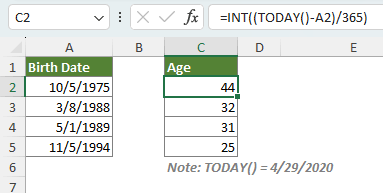Notes:

• To get someone's age at a certain date, replace the TODAY() function in the formula with that specific date. For instance, if the particular date is in cell B2, use the formula below:
``=INT((B2-A2)/365)``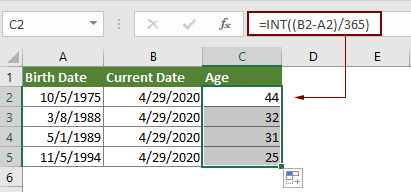• Using this formula in Excel, which divides the difference in days between two dates by 365, usually yields accurate results, but it's not perfect. It may inaccurately calculate ages around leap years or for those born on February 29. An alternative is to divide by 365.25 to account for leap years, but this can also lead to errors, especially for ages not spanning a leap year. While this method is generally effective, it's not ideal for precise calculations. The following formulas introduce specialized functions that provide flawless age calculations in all scenarios.

##### Convert birthdate to age with the YEARFRAC function

The YEARFRAC function is more accurate for calculating age from a date of birth because it considers the exact number of days in each year, including leap years. By setting the third argument, basis, to 1, YEARFRAC uses the actual day count in its calculation, ensuring precision, especially in cases involving leap years.

In an empty cell where you want the age calculated, enter the formula below and press Enter. To replicate this calculation for other data, drag the fill handle of the result cell downward.

``=ROUNDDOWN(YEARFRAC(A2, TODAY(), 1), 0)``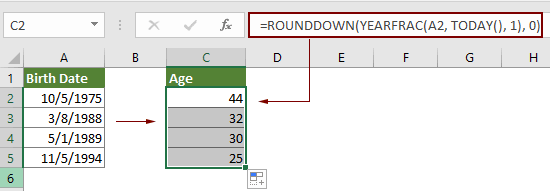##### Convert birthdate to age with the DATEDIF function

The DATEDIF function in Excel can be used to calculate age from a date of birth. By using "y" in the unit argument of the function, it calculates the number of complete years between two dates.

Select an empty cell to display the age, input the formula provided below, and hit the Enter key. Then, click on the cell with the result and drag its fill handle downwards to apply the formula to other cells and obtain all results.

``=DATEDIF(A2,NOW(),"y")``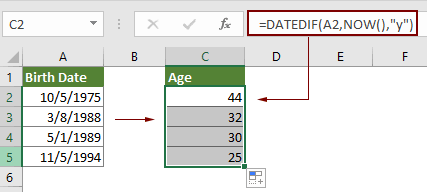Note: In the above DATEDIF formula, "y" calculates the complete years from the date in cell A2 to today. You can substitute "y" with "m", "d", "md", "ym", or "yd" to calculate different aspects of the date difference:

• "M": The number of complete months in the given period.
• "D": The number of days in the given period.
• "MD": The difference between the days of the given two dates. The months and years of the dates are ignored.
• "YM": The difference between the months of the given two dates. The days and years of the dates are ignored
• "YD": The difference between the days of the given two dates. The years of the dates are ignored.

##### Display age in Year + Month + Day format with the DATEDIF function

To calculate age in the format of Year + Month + Day, combine three DATEDIF formulas in Excel, each with a different unit argument.

Select an empty cell to display the age, input the formula provided below, and hit the Enter key. Then, click on the cell with the result and drag its fill handle downwards to apply the formula to other cells and obtain all results.

``=DATEDIF(A2,TODAY(),"Y") & " Years, " & DATEDIF(A2,TODAY(),"YM") & " Months, " & DATEDIF(A2,TODAY(),"MD") & " Days"``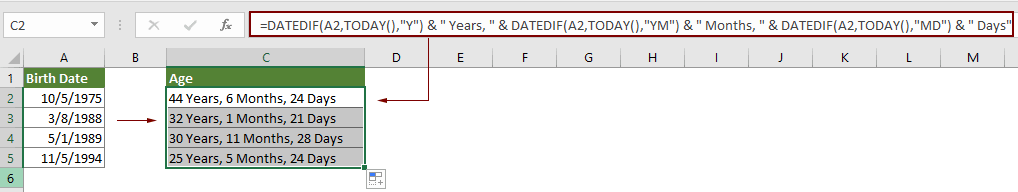#### Easily calculate age from date of birth without remembering formulas

Kutools for Excel's Date & Time Helper feature simplifies age calculations, allowing you to easily convert birthdates to age with just a few clicks, without the need to remember formulas. It offers the flexibility to calculate age up to the current day or a specific date, and provides results in various formats like years, months, weeks, days, or a combination of years + months + days. This user-friendly tool streamlines the process, making it quick and accessible for all Excel users.

1. Select a blank cell to display the age. Then click Kutools > Formula Helper > Date & Time Helper.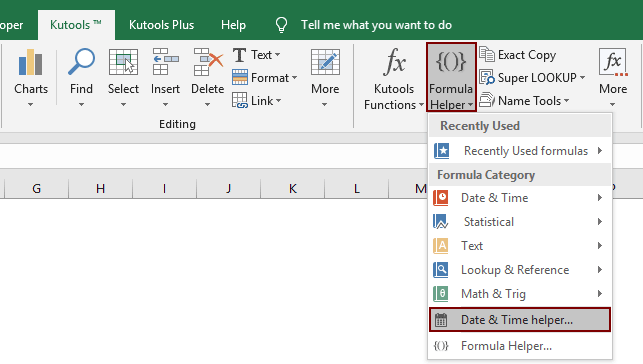2. In the Date & Time Helper dialog box, please configure as follows.
1. Go to the Age tab;
2. In the Date of Birth box, select the cell containing the birth date you wish to calculate the age from;
3. Choose the Today option in the To section;
4. Choose the desired age output format, like years, months, days, or a combination, from the Output result type drop-down list;
5. Click the OK button.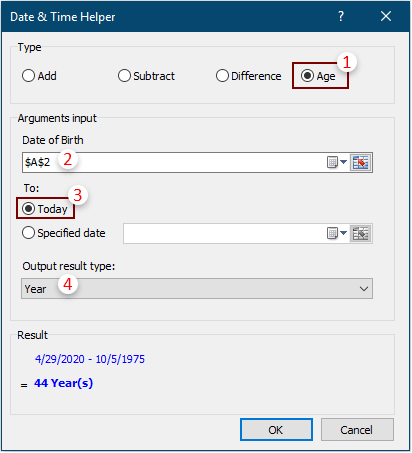Result

Then the age is populated in the selected cell. Select the result cell and then drag its fill handle all the way down to get all ages.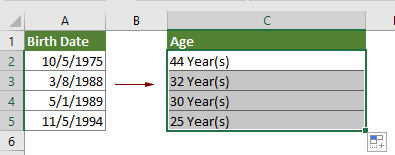Notes:

• To display the age in the Year + Month + Day format, select Year + Month + Day from the Output result type drop-down list. The result will appear as illustrated in the screenshot below. Additionally, this feature supports displaying age in months, weeks, or days, catering to your specific requirements.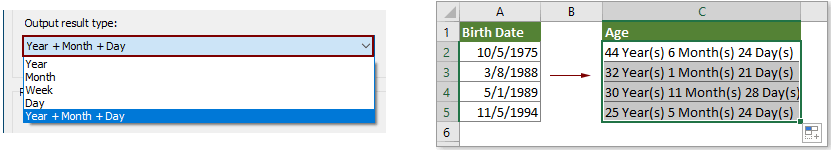• Want to access the Date & Time Helper feature? Download Kutools for Excel now! Beyond this, Kutools boasts a myriad of 300+ other features and offers a 30-day free trial. Don't wait, give it a try today!

### Best Office Productivity Tools

 Popular Features: Find, Highlight or Identify Duplicates   |  Delete Blank Rows   |  Combine Columns or Cells without Losing Data   |   Round without Formula ... Super Lookup: Multiple Criteria VLookup  |   Multiple Value VLookup  |   VLookup Across Multiple Sheets   |   Fuzzy Lookup .... Advanced Drop-down List: Quickly Create Drop Down List   |  Dependent Drop Down List   |  Multi-select Drop Down List .... Column Manager: Add a Specific Number of Columns  |  Move Columns  |  Toggle Visibility Status of Hidden Columns  |  Compare Ranges & Columns ... Featured Features: Grid Focus   |  Design View   |   Big Formula Bar   |  Workbook & Sheet Manager   |  Resource Library (Auto Text)   |  Date Picker   |  Combine Worksheets   |  Encrypt/Decrypt Cells   |  Send Emails by List   |  Super Filter   |   Special Filter (filter bold/italic/strikethrough...) ... Top 15 Toolsets:  12 Text Tools (Add Text, Remove Characters, ...)   |   50+ Chart Types (Gantt Chart, ...)   |   40+ Practical Formulas (Calculate age based on birthday, ...)   |   19 Insertion Tools (Insert QR Code, Insert Picture from Path, ...)   |   12 Conversion Tools (Numbers to Words, Currency Conversion, ...)   |   7 Merge & Split Tools (Advanced Combine Rows, Split Cells, ...)   |   ... and more

Supercharge Your Excel Skills with Kutools for Excel, and Experience Efficiency Like Never Before. Kutools for Excel Offers Over 300 Advanced Features to Boost Productivity and Save Time.  Click Here to Get The Feature You Need The Most...#### Office Tab Brings Tabbed interface to Office, and Make Your Work Much Easier

• Enable tabbed editing and reading in Word, Excel, PowerPoint, Publisher, Access, Visio and Project.
• Open and create multiple documents in new tabs of the same window, rather than in new windows.
• Increases your productivity by 50%, and reduces hundreds of mouse clicks for you every day!No ratings yet. Be the first to rate!
This comment was minimized by the moderator on the site
I'm creating automated age cell by using the following formula =IFDATED(C12,TODAY(),"Y") but when I drag the cell down to empty cells without DOB, calculation is been made for empty cells, what can I do to empty cell not to calculate except if DOB in not typed?
This comment was minimized by the moderator on the site
Hi there,

You mean DATEDIF, right?
You can add an IF function as shown below: =IF(C12="","",DATEDIF(C12,TODAY(),"Y"))

Amanda
This comment was minimized by the moderator on the site
Thank you very much, the formula work.
This comment was minimized by the moderator on the site
A VERY DIFFICULT INSTRUCTIONS! NOT GIVING THE RIGHT RESULT
This comment was minimized by the moderator on the site
Hi, sorry that the methods list in the article did not help you. But can you tell what are your data and what method did you use, so the result went wrong?
This comment was minimized by the moderator on the site
How to convert age to date of birth
This comment was minimized by the moderator on the site
Hi Catherine,

thank you so much for your formula! I am a related service provider for many students with various ages in a school setting. thanks to your formula, the student's age is in front of me during each session, and I am able to adjust session goals appropriately.
This comment was minimized by the moderator on the site
thanks you so much very very good formula
This comment was minimized by the moderator on the site
Just wanted to say THANK YOU!
This comment was minimized by the moderator on the site
Do not show the Detedif Formula in my Excel
What to do Know?
This comment was minimized by the moderator on the site
if I have their id number how to get their age example their id no consist first 6digit is date of birth

example 830901056252 , 830901 is date of birth.

how to take calculate their age
This comment was minimized by the moderator on the site
Dear elsie,
Please try this formula: =DATEDIF(DATE(IF(LEFT(A2,2)>TEXT(TODAY(),"YY"),"19"&LEFT(A2,2),"20"&LEFT(A2,2)),MID(A2,3,2),MID(A2,5,2)),TODAY(),"y"). A2 is the cell contains the ID number you want to calculate the age based on.
This comment was minimized by the moderator on the site
Thanks soooooo much dear....!
This comment was minimized by the moderator on the site
please do i calculate the birth date from age
This comment was minimized by the moderator on the site
Good Day,
This formula =DATE(YEAR(TODAY())-A1,MONTH(TODAY()),DAY(TODAY())) can help you to calculate the birthday from a given age based on today's date.
There are no comments posted here yet